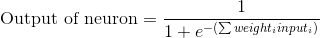# Sigmoid function

sigmoid function is a mathematical function having a characteristic “S”-shaped curve or sigmoid curve. It is used in neural networks as an activation function, defining the output of a node given a set of inputs.

The Sigmoid function often refers to the special case of the logistic function shown in the first figure and defined by the formula:The graph representing this function forms an “S” shape from R to ]0;1[The sigmoid function has a first derivative that is always non-negative and forms a bell shape.

Read more on the sigmoid function on Wikipedia.

## The use of the sigmoid function in neural networks

The sigmoid function is used to program neural networks as an activation function. It is a standard function in a neural net node (a “neuron”), used to normalize the sum of data inputs after applying weights.

It ensures the output of the node is always in the interval ]0;1[, while also signifying high confidence for large positive or negative numbers.

In practice the sigmoid function used in neural nets has the following formula:The sigmoid function derivative is used in the training period to modify weights applied to the training data, during the process of gradient descent.

Read more on the use of the sigmoid function in the post on programming a simple neural network or review the video on principles of neural nets.

« Back to Glossary Index